How Cheenta works to ensure student success?
Explore the Back-Story

# Circles and semi-circles| AMC 8, 2010|Problem 23Try this beautiful problem from Geometry based on Ratio of the area of circle and semi-circles.

## Area of circles and semi-circles - AMC-8, 2010 - Problem 23

Semicircles POQ and ROS pass through the center O. What is the ratio of the combined areas of the two semicircles to the area of circle O?

••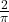•### Key Concepts

Geometry

Circle

co-ordinate geometry

Answer:AMC-8 (2010) Problem 23

Pre College Mathematics

## Try with Hints

Find the radius of the circle

Can you now finish the problem ..........

Join O and Q

can you finish the problem........

The co-ordinate of Q is (1,1), So OB=1 and BQ=1

By the Pythagorean Theorem, the radius of the larger circle i.e OQ=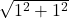=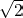.

Therefore the area of the larger circle be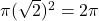Now for the semicircles, radius OB=OC=1(as co-ordinate of P=(1,1) and S=(1,-1))

So, the area of the two semicircles is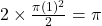Finally, the ratio of the combined areas of the two semicircles to the area of circle O is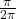=## Subscribe to Cheenta at Youtube

Try this beautiful problem from Geometry based on Ratio of the area of circle and semi-circles.

## Area of circles and semi-circles - AMC-8, 2010 - Problem 23

Semicircles POQ and ROS pass through the center O. What is the ratio of the combined areas of the two semicircles to the area of circle O?

•••### Key Concepts

Geometry

Circle

co-ordinate geometry

Answer:AMC-8 (2010) Problem 23

Pre College Mathematics

## Try with Hints

Find the radius of the circle

Can you now finish the problem ..........

Join O and Q

can you finish the problem........

The co-ordinate of Q is (1,1), So OB=1 and BQ=1

By the Pythagorean Theorem, the radius of the larger circle i.e OQ==.

Therefore the area of the larger circle beNow for the semicircles, radius OB=OC=1(as co-ordinate of P=(1,1) and S=(1,-1))

So, the area of the two semicircles isFinally, the ratio of the combined areas of the two semicircles to the area of circle O is=## Subscribe to Cheenta at Youtube

This site uses Akismet to reduce spam. Learn how your comment data is processed.

### Knowledge Partner